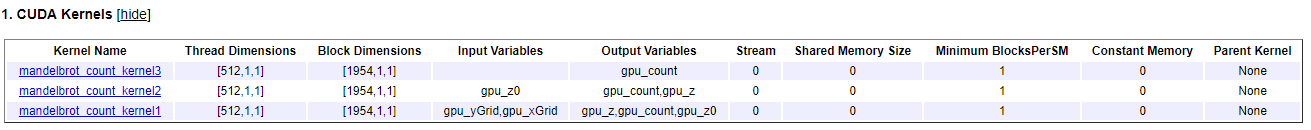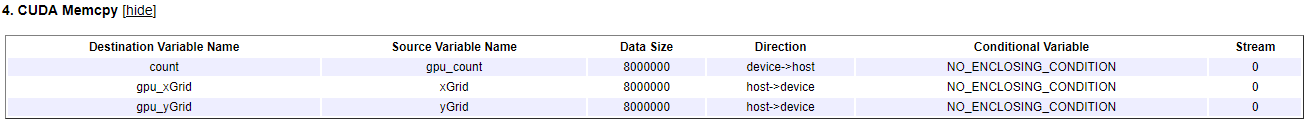## Generating a GPU Code Metrics Report for Code Generated from MATLAB Code

The GPU static code metrics report contains the results of static analysis of the generated CUDA® code, including information on the generated CUDA kernels, thread and block dimensions, memory usage and other statistics. To produce a static code metrics report, you must use GPU Coder™ to generate standalone CUDA code and produce a code generation report. See Code Generation Reports.

By default, static code metrics analysis does not run at code generation time. Instead, if and when you want to run the analysis and view the results, click GPU Code Metrics on the Summary tab of the code generation report.

### Example GPU Code Metrics Report

This example runs GPU static code metrics analysis and examines a static code metrics report.

Create a MATLAB® function called `mandelbrot_count.m` with the following lines of code. This code is a vectorized MATLAB implementation of the Mandelbrot set. For every point `(xGrid,yGrid)` in the grid, it calculates the iteration index `count` at which the trajectory defined by the equation reaches a distance of `2` from the origin. It then returns the natural logarithm of `count`, which is used generate the color coded plot of the Mandelbrot set.

```function count = mandelbrot_count(maxIterations,xGrid,yGrid) % Add kernelfun pragma to trigger kernel creation coder.gpu.kernelfun; % mandelbrot computation z0 = xGrid + 1i*yGrid; count = ones(size(z0)); z = z0; for n = 0:maxIterations z = z.*z + z0; inside = abs(z)<=2; count = count + inside; end count = log(count); ```

Create sample data with the following lines of code. The code generates a 1000 x 1000 grid of real parts (x) and imaginary parts (y) between the limits specified by `xlim` and `ylim`.

```maxIterations = 500; gridSize = 1000; xlim = [-0.748766713922161,-0.748766707771757]; ylim = [0.123640844894862,0.123640851045266]; x = linspace(xlim(1),xlim(2),gridSize); y = linspace(ylim(1),ylim(2),gridSize); [xGrid,yGrid] = meshgrid(x,y); ```

Enable production of a code generation report by using a configuration object for standalone code generation (static library, dynamically linked library, or executable program).

```cfg = coder.gpuConfig('dll'); cfg.GenerateReport = true; cfg.MATLABSourceComments = true; cfg.GpuConfig.CompilerFlags = '--fmad=false'; ```

Note

The `--fmad=false` flag when passed to the `nvcc`, instructs the compiler to disable Floating-Point Multiply-Add (FMAD) optimization. This option is set to prevent numerical mismatch in the generated code because of architectural differences in the CPU and the GPU. For more information, see Numerical Differences Between CPU and GPU.

Alternatively, use the `codegen` `-report` option.

Generate code by using `codegen`. Specify the type of the input argument by providing an example input with the `-args` option. Specify the configuration object by using the `-config ` option.

```codegen -config cfg -args {maxIterations,xGrid,yGrid} mandelbrot_count ```

To open the code generation report, click View report.

To run the static code metrics analysis and view the code metrics report, on the Summary tab of the code generation report, click GPU Code Metrics.

### Explore the code metrics report

1. To see the information on the generated CUDA kernels, click CUDA Kernels.• Kernel Name contains the list of generated CUDA kernels. By default, GPU Coder prepends the kernel name with the name of the entry-point function.

• Thread Dimensions is an array of the form `[Tx,Ty,Tz]` that identifies the number of threads in the block along dimensions `x`, `y`, and `z`.

• Block Dimensions is an array of the form `[Bx,By,1]` is an array that defines the number of blocks in the grid along dimensions `x` and `y` (`z` not used).

• Shared Memory Size and Constant Memory columns provide metrics on the shared and constant memory space usage in the generated code.

• Minimum BlocksPerSM is the minimum number of blocks per streaming multiprocessor and indicates the number of blocks with which to launch the kernels.

To navigate from the report to the generated kernel code, click a kernel name.

2. To see the variables that have memory allocated on the GPU device, go to the CUDA Malloc section.3. To view information on the `cudaMemCpy` calls in the generated code, click CUDA Memcpy.### Limitations

• If you have the Embedded Coder® product, the code configuration object contains the `GenerateCodeMetricsReport` property to enable static metric report generation at compile time. GPU Coder does not honor this setting and has no effect during code generation.